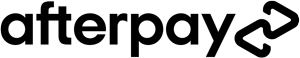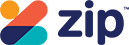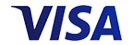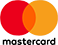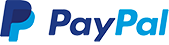Grid ListWAS \$49.95 NOW \$24.95
Increase value Decrease valueWAS \$49.95 NOW \$24.95
Increase value Decrease valueWAS \$49.95 NOW \$24.95
Increase value Decrease valueWAS \$49.95 NOW \$24.95
Increase value Decrease valueWAS \$49.95 NOW \$24.95
Increase value Decrease valueWAS \$49.95 NOW \$24.95
Increase value Decrease value\$24.95
Increase value Decrease value\$24.95
Increase value Decrease value\$24.95
Increase value Decrease value\$24.95
Increase value Decrease value\$24.95
Increase value Decrease value\$9.95
Increase value Decrease value